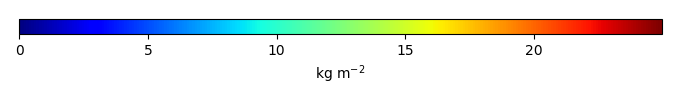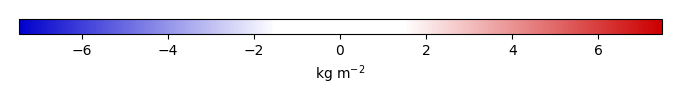# Mean State

Period Mean (original grids) [Pg]
Model Period Mean (intersection) [Pg]
Model Period Mean (complement) [Pg]
Benchmark Period Mean (intersection) [Pg]
Benchmark Period Mean (complement) [Pg]
Bias [kg m-2]
Bias Score 
Spatial Distribution Score 
Overall Score 
Benchmark [-] 32.8
CRUNCEPv7 [-] 42.9 43.3 0.233 32.8 0.0526 2.86 0.65 0.86 0.76
GSWP3v1 [-] 38.5 38.7 0.241 32.8 0.0526 1.73 0.72 0.93 0.82
WATCH [-] 38.8 39.5 0.235 32.8 0.0526 1.91 0.73 0.94 0.84
Period Mean (original grids) [Pg]
Model Period Mean (intersection) [Pg]
Model Period Mean (complement) [Pg]
Benchmark Period Mean (intersection) [Pg]
Benchmark Period Mean (complement) [Pg]
Bias [kg m-2]
Bias Score 
Spatial Distribution Score 
Overall Score 
Benchmark [-] 8.35
CRUNCEPv7 [-] 3.54 2.95 0.191 8.34 0.0114 -0.934 0.50 0.66 0.58
GSWP3v1 [-] 3.24 2.69 0.201 8.34 0.0114 -0.977 0.48 0.62 0.55
WATCH [-] 6.17 5.28 0.220 8.34 0.0114 -0.518 0.57 0.88 0.72
Period Mean (original grids) [Pg]
Model Period Mean (intersection) [Pg]
Model Period Mean (complement) [Pg]
Benchmark Period Mean (intersection) [Pg]
Benchmark Period Mean (complement) [Pg]
Bias [kg m-2]
Bias Score 
Spatial Distribution Score 
Overall Score 
Benchmark [-] 10.6
CRUNCEPv7 [-] 17.1 16.4 0.388 10.6 0.0246 0.803 0.51 0.51 0.51
GSWP3v1 [-] 12.3 11.7 0.363 10.6 0.0246 0.299 0.50 0.56 0.53
WATCH [-] 20.7 20.0 0.385 10.6 0.0246 1.21 0.45 0.45 0.45
Period Mean (original grids) [Pg]
Model Period Mean (intersection) [Pg]
Model Period Mean (complement) [Pg]
Benchmark Period Mean (intersection) [Pg]
Benchmark Period Mean (complement) [Pg]
Bias [kg m-2]
Bias Score 
Spatial Distribution Score 
Overall Score 
Benchmark [-] 4.69
CRUNCEPv7 [-] 5.06 5.01 0.0720 4.67 0.0241 0.237 0.60 0.86 0.73
GSWP3v1 [-] 4.17 4.12 0.0636 4.67 0.0241 -0.0436 0.58 0.80 0.69
WATCH [-] 5.34 5.30 0.0697 4.67 0.0241 0.336 0.64 0.83 0.74
Period Mean (original grids) [Pg]
Model Period Mean (intersection) [Pg]
Model Period Mean (complement) [Pg]
Benchmark Period Mean (intersection) [Pg]
Benchmark Period Mean (complement) [Pg]
Bias [kg m-2]
Bias Score 
Spatial Distribution Score 
Overall Score 
Benchmark [-] 27.8
CRUNCEPv7 [-] 49.4 47.3 1.89 26.8 0.921 11.4 0.47 0.91 0.69
GSWP3v1 [-] 39.9 38.2 1.51 26.8 0.921 7.87 0.61 0.92 0.76
WATCH [-] 38.9 37.1 1.55 26.8 0.921 7.57 0.63 0.88 0.76
Period Mean (original grids) [Pg]
Model Period Mean (intersection) [Pg]
Model Period Mean (complement) [Pg]
Benchmark Period Mean (intersection) [Pg]
Benchmark Period Mean (complement) [Pg]
Bias [kg m-2]
Bias Score 
Spatial Distribution Score 
Overall Score 
Benchmark [-] 43.5
CRUNCEPv7 [-] 73.2 71.7 0.369 43.5 0.0288 3.33 0.50 0.55 0.52
GSWP3v1 [-] 52.5 51.4 0.326 43.5 0.0288 1.03 0.49 0.63 0.56
WATCH [-] 49.8 48.8 0.265 43.5 0.0288 0.704 0.67 0.93 0.80
Period Mean (original grids) [Pg]
Model Period Mean (intersection) [Pg]
Model Period Mean (complement) [Pg]
Benchmark Period Mean (intersection) [Pg]
Benchmark Period Mean (complement) [Pg]
Bias [kg m-2]
Bias Score 
Spatial Distribution Score 
Overall Score 
Benchmark [-] 32.0
CRUNCEPv7 [-] 36.1 36.1 0.765 31.9 0.0804 0.598 0.50 0.75 0.63
GSWP3v1 [-] 31.6 31.8 0.632 31.9 0.0804 0.262 0.61 0.86 0.73
WATCH [-] 33.2 33.1 0.669 31.9 0.0804 0.336 0.65 0.89 0.77
Period Mean (original grids) [Pg]
Model Period Mean (intersection) [Pg]
Model Period Mean (complement) [Pg]
Benchmark Period Mean (intersection) [Pg]
Benchmark Period Mean (complement) [Pg]
Bias [kg m-2]
Bias Score 
Spatial Distribution Score 
Overall Score 
Benchmark [-] 21.1
CRUNCEPv7 [-] 25.4 24.1 0.687 20.9 0.146 1.53 0.54 0.74 0.64
GSWP3v1 [-] 24.8 23.3 0.610 20.9 0.146 1.28 0.60 0.82 0.71
WATCH [-] 25.5 24.3 0.604 20.9 0.146 1.42 0.60 0.83 0.72
Period Mean (original grids) [Pg]
Model Period Mean (intersection) [Pg]
Model Period Mean (complement) [Pg]
Benchmark Period Mean (intersection) [Pg]
Benchmark Period Mean (complement) [Pg]
Bias [kg m-2]
Bias Score 
Spatial Distribution Score 
Overall Score 
Benchmark [-] 3.81
CRUNCEPv7 [-] 8.81 9.19 0.106 3.81 0.000319 1.63 0.46 0.68 0.57
GSWP3v1 [-] 6.88 7.17 0.0941 3.81 0.000319 1.03 0.51 0.75 0.63
WATCH [-] 5.00 5.20 0.0595 3.81 0.000319 0.445 0.68 0.91 0.80
Period Mean (original grids) [Pg]
Model Period Mean (intersection) [Pg]
Model Period Mean (complement) [Pg]
Benchmark Period Mean (intersection) [Pg]
Benchmark Period Mean (complement) [Pg]
Bias [kg m-2]
Bias Score 
Spatial Distribution Score 
Overall Score 
Benchmark [-] 9.16
CRUNCEPv7 [-] 11.5 10.5 0.819 8.87 0.289 0.811 0.58 0.78 0.68
GSWP3v1 [-] 11.0 10.0 0.829 8.87 0.289 0.760 0.60 0.81 0.71
WATCH [-] 11.8 10.8 0.800 8.87 0.289 0.850 0.62 0.82 0.72
Period Mean (original grids) [Pg]
Model Period Mean (intersection) [Pg]
Model Period Mean (complement) [Pg]
Benchmark Period Mean (intersection) [Pg]
Benchmark Period Mean (complement) [Pg]
Bias [kg m-2]
Bias Score 
Spatial Distribution Score 
Overall Score 
Benchmark [-] 12.0
CRUNCEPv7 [-] 26.8 25.7 0.646 12.0 0.0529 3.42 0.44 0.26 0.35
GSWP3v1 [-] 18.2 17.4 0.537 12.0 0.0529 1.77 0.47 0.35 0.41
WATCH [-] 13.3 12.6 0.451 12.0 0.0529 0.862 0.51 0.53 0.52
Period Mean (original grids) [Pg]
Model Period Mean (intersection) [Pg]
Model Period Mean (complement) [Pg]
Benchmark Period Mean (intersection) [Pg]
Benchmark Period Mean (complement) [Pg]
Bias [kg m-2]
Bias Score 
Spatial Distribution Score 
Overall Score 
Benchmark [-] 368.
CRUNCEPv7 [-] 589. 578. 11.2 363. 4.64 2.37 0.51 0.68 0.60
GSWP3v1 [-] 487. 477. 9.77 363. 4.64 1.46 0.57 0.78 0.68
WATCH [-] 505. 496. 9.63 363. 4.64 1.60 0.60 0.81 0.70
Period Mean (original grids) [Pg]
Model Period Mean (intersection) [Pg]
Model Period Mean (complement) [Pg]
Benchmark Period Mean (intersection) [Pg]
Benchmark Period Mean (complement) [Pg]
Bias [kg m-2]
Bias Score 
Spatial Distribution Score 
Overall Score 
Benchmark [-] 1.24
CRUNCEPv7 [-] 1.68 1.27 0.401 1.23 0.00783 0.0633 0.49 0.67 0.58
GSWP3v1 [-] 1.55 1.22 0.341 1.23 0.00783 0.0659 0.51 0.74 0.62
WATCH [-] 2.27 1.81 0.433 1.23 0.00783 0.204 0.51 0.73 0.62
Period Mean (original grids) [Pg]
Model Period Mean (intersection) [Pg]
Model Period Mean (complement) [Pg]
Benchmark Period Mean (intersection) [Pg]
Benchmark Period Mean (complement) [Pg]
Bias [kg m-2]
Bias Score 
Spatial Distribution Score 
Overall Score 
Benchmark [-] 5.62
CRUNCEPv7 [-] 16.4 16.0 0.288 5.59 0.0265 1.94 0.30 0.36 0.33
GSWP3v1 [-] 14.5 14.1 0.228 5.59 0.0265 1.60 0.34 0.40 0.37
WATCH [-] 11.4 11.0 0.209 5.59 0.0265 1.04 0.45 0.55 0.50
Period Mean (original grids) [Pg]
Model Period Mean (intersection) [Pg]
Model Period Mean (complement) [Pg]
Benchmark Period Mean (intersection) [Pg]
Benchmark Period Mean (complement) [Pg]
Bias [kg m-2]
Bias Score 
Spatial Distribution Score 
Overall Score 
Benchmark [-] 8.21
CRUNCEPv7 [-] 11.9 11.1 0.518 8.09 0.122 2.42 0.50 0.50 0.50
GSWP3v1 [-] 10.2 9.46 0.474 8.09 0.122 1.74 0.54 0.60 0.57
WATCH [-] 10.8 10.1 0.449 8.09 0.122 2.00 0.54 0.62 0.58
Period Mean (original grids) [Pg]
Model Period Mean (intersection) [Pg]
Model Period Mean (complement) [Pg]
Benchmark Period Mean (intersection) [Pg]
Benchmark Period Mean (complement) [Pg]
Bias [kg m-2]
Bias Score 
Spatial Distribution Score 
Overall Score 
Benchmark [-] 14.8
CRUNCEPv7 [-] 17.8 17.4 0.231 14.8 0.0147 1.04 0.63 0.72 0.67
GSWP3v1 [-] 12.0 11.7 0.182 14.8 0.0147 -0.425 0.65 0.87 0.76
WATCH [-] 13.9 13.6 0.173 14.8 0.0147 0.0172 0.70 0.91 0.80
Period Mean (original grids) [Pg]
Model Period Mean (intersection) [Pg]
Model Period Mean (complement) [Pg]
Benchmark Period Mean (intersection) [Pg]
Benchmark Period Mean (complement) [Pg]
Bias [kg m-2]
Bias Score 
Spatial Distribution Score 
Overall Score 
Benchmark [-] 20.1
CRUNCEPv7 [-] 56.8 56.7 0.307 19.8 0.219 7.50 0.30 0.63 0.47
GSWP3v1 [-] 43.6 43.6 0.247 19.8 0.219 4.91 0.40 0.72 0.56
WATCH [-] 38.9 38.8 0.228 19.8 0.219 3.98 0.46 0.81 0.64
Period Mean (original grids) [Pg]
Model Period Mean (intersection) [Pg]
Model Period Mean (complement) [Pg]
Benchmark Period Mean (intersection) [Pg]
Benchmark Period Mean (complement) [Pg]
Bias [kg m-2]
Bias Score 
Spatial Distribution Score 
Overall Score 
Benchmark [-] 8.04
CRUNCEPv7 [-] 3.49 3.46 0.0449 7.99 0.0515 -0.996 0.52 0.65 0.59
GSWP3v1 [-] 3.04 3.04 0.0352 7.99 0.0515 -1.10 0.51 0.49 0.50
WATCH [-] 3.35 3.34 0.0401 7.99 0.0515 -1.04 0.51 0.51 0.51
Period Mean (original grids) [Pg]
Model Period Mean (intersection) [Pg]
Model Period Mean (complement) [Pg]
Benchmark Period Mean (intersection) [Pg]
Benchmark Period Mean (complement) [Pg]
Bias [kg m-2]
Bias Score 
Spatial Distribution Score 
Overall Score 
Benchmark [-] 88.9
CRUNCEPv7 [-] 152. 151. 0.431 88.8 0.0655 7.77 0.55 0.89 0.72
GSWP3v1 [-] 129. 128. 0.373 88.8 0.0655 5.00 0.65 0.94 0.80
WATCH [-] 144. 144. 0.361 88.8 0.0655 6.83 0.58 0.91 0.74
Period Mean (original grids) [Pg]
Model Period Mean (intersection) [Pg]
Model Period Mean (complement) [Pg]
Benchmark Period Mean (intersection) [Pg]
Benchmark Period Mean (complement) [Pg]
Bias [kg m-2]
Bias Score 
Spatial Distribution Score 
Overall Score 
Benchmark [-] 9.09
CRUNCEPv7 [-] 21.7 21.8 0.315 9.08 0.0128 2.16 0.42 0.39 0.40
GSWP3v1 [-] 21.8 21.8 0.283 9.08 0.0128 2.13 0.32 0.30 0.31
WATCH [-] 23.9 23.9 0.260 9.08 0.0128 2.44 0.32 0.36 0.34
Period Mean (original grids) [Pg]
Model Period Mean (intersection) [Pg]
Model Period Mean (complement) [Pg]
Benchmark Period Mean (intersection) [Pg]
Benchmark Period Mean (complement) [Pg]
Bias [kg m-2]
Bias Score 
Spatial Distribution Score 
Overall Score 
Benchmark [-] 2.45
CRUNCEPv7 [-] 5.25 5.16 0.145 2.40 0.0496 1.70 0.37 0.29 0.33
GSWP3v1 [-] 6.26 6.15 0.140 2.40 0.0496 1.92 0.35 0.32 0.34
WATCH [-] 5.86 5.78 0.127 2.40 0.0496 1.71 0.38 0.40 0.39

# Temporally integrated period mean

BENCHMARK MEANMODEL MEANBIASBIAS SCORESPATIAL TAYLOR DIAGRAMMODEL COLORS# Spatially integrated regional mean

MODEL COLORS# All Models

BenchmarkCRUNCEPv7GSWP3v1WATCH